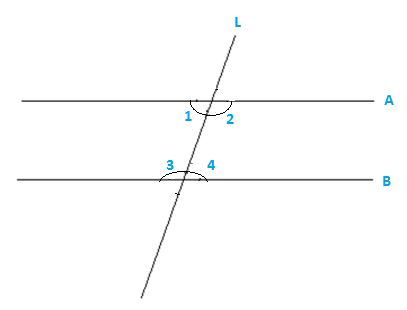### NCERT SOLUTIONS for Class 9 Math Chapter – 5 Exercise – 5.2

NCERT Solutions For Class 9 Math Chapter – 5 Exercise – 5.2

Q1. How would you rewrite Euclid fifth postulate so that it would be easier to understand?

Solution:-

The fifth postulates is about parallel lines.

When two or more never intersect each other in a plane and perpendicular distance between them is always constant then they are said to be parallel lines

Two facts of the postulates:

• If P doesn’t lie on I then we can draw a line through P which will be parallel to the line L.
• There will be only one line can be drawn through P which is parallel to the line L.

Q2.

Does Euclid fifth postulate imply the existence of parallel lines? Explain.

Solution:-

Yes , Euclid fifth postulate imply the existence of parallel lines.

If the sum of the interior  angles will be equal to sum of the two right angles then two lines will not meet each other on either sides and therefore the will be parallel to each other.M and N will be parallel if

∟1 + ∟3 = 1800

Or ∟3 + ∟4 = 1800